# Dynamic Simulation of a Solar Electric Driven Heat Pump for an Office Building Located in Southern Italy

Dynamic Simulation of a Solar Electric Driven Heat Pump for an Office Building Located in Southern Italy

C. RoselliM. Sasso F. Tariello

Università Degli Studi Del Sannio, Dipartimento di Ingegneria, Piazza Roma 21, Benevento, Italy

Corresponding Author Email:
carlo.roselli@unisannio.it
Page:
S496-S504
|
DOI:
https://doi.org/10.18280/ijht.34S243
|
Accepted:
|
Published:
31 October 2016
| Citation

OPEN ACCESS

Abstract:

The paper investigates the introduction of a solar electric driven heat pump for an office building, placed in southern Italy, with low energy demand in comparison with the most common type of Italian buildings. Dynamic simulations to evaluate energy, environmental and economic performance of the proposed system, varying photovoltaic peak powers (4500 W - 7500 W), tilt angles (20° - 50°), electricity and natural gas unit prices, are carried out. To meet heating and cooling demand a heat pump driven by electricity available from solar photovoltaic panels is here considered. The system based on solar electric driven heat pump shows a saving in terms of primary energy and equivalent CO2 emission reduction up to about 81 % if compared to the reference conventional system based on a boiler fueled by natural gas and an electric-driven chiller. The results highlight that the solar based energy conversion system is more interesting when the electric energy and natural gas unit prices are high and government incentives are available.

Keywords:

Solar electric heat pump, Dynamic simulation.

1. Introduction

In European Union, considering the period 2011-2013, the energy consumption in the civil sector, with respect to the total final energy use, increased from 37.4 % to 40.6 % , surpassing other sectors such as industry, which reaches only the 25.1% in 2013 . This trend has determined an increasing interest in the reduction of primary energy consumption and greenhouse gas emissions in the residential and tertiary sectors due to typical energy demands (space heating and cooling, domestic hot water) of a building. Different paths could be followed in order to achieve this goal and the most common technologies and techniques are:

• building renovation through interventions on opaque and transparent envelope aiming to reduce transmittance;
• installing fossil fuel based energy conversion systems characterized by high efficiency, such as condensing boiler, cogeneration system, gas engine-driven heat pump (GHP), ground source heat pump (GSHP);
• introducing renewable energy system, such as solar heating and cooling plant using solar thermal collectors (flat plat, evacuated tube) and thermally-activated heat pump;
• solar systems driven by electricity : solar photovoltaic (PV) system activating a reversible electric heat pump (EHP) used to meet space heating and cooling demand;
• electric heat pump interacting with solar thermal collector: thermal energy delivered by solar collectors is used to activate an EHP at lower evaporating temperature ;
• a combination of renewable-based and high energy conversion efficiency devices .

Particularly interesting seems to be technologies exploiting solar source for heating and cooling purpose. In the last years great interest was generated by solar heating and cooling (SHC) systems based on thermally-activated refrigeration devices  such as adsorption  and absorption heat pumps , evaporative and ejector cooling systems , HVAC (Heating and Ventilation Air Conditioning) desiccant-based systems . These systems can guarantee interesting primary energy saving up to 67 %, as stated by Ref.  even if investment costs are too high to make the technology suitable for the actual market . A different way to exploit solar energy is the introduction of a photovoltaic system interacting with a reversible heat pump. In  the authors perform a theoretical-experimental analysis on a reversible air to water heat pump interacting with a solar system based on PV panels with a peak power of 2.88 kW and electric batteries with a capacity of 250 Ah. The air to water heat pump, with a nominal thermal power of 6 kW, heats a laboratory, located in Spain, supplying hot water to radiant floor. Hartmann et al. in  compare solar thermal and solar electric cooling systems for a typical small office building in two different European locations (Freiburg and Madrid) by means of TRNSYS. The authors investigate through energy and economic indices, varying solar collecting area, the performance of the systems. Large collector areas lead up to 40 % for Freiburg and 60 % for Madrid of primary energy saving with the solar thermal system as well as with the solar PV system. The economic results show that PV based system is mature for market while adsorption based system needs further improvements in performance and a strong reduction in terms of investment cost aiming the technology achieving an interesting diffusion. The paper reported in Ref.  analyzes a system based on 3.7 kW PV plant interacting with a GSHP installed in a residential building, a single-family house of 160 m2 and a volume of about 450 m3. The study aims to optimize the system increasing self-consumption of the energy available from PV plant. The results show that the level of interaction with the electric grid is quite high and the introduction of a minimum storage capacity (200-400 kWh) is required to reduce electricity sent to the grid. Reda et al. in  analyze solar-based technologies, PV modules and solar thermal collectors, interacting with a GSHP for different Italian localities by means of TRNSYS software. The proposed system leads to low energy consumption mainly in locations characterized by high solar irradiance where the use of small PV and solar thermal systems allow interesting energy savings.

The previous literature shows that existing works are focused on experimental or simulation analysis of solar heating and cooling plants consisting of electric heat pump interacting with a PV field. In this paper an energy, environmental and economic analysis is carried out on a PV plant interacting with an EHP that meets space heating and cooling demand of an office building. This building respects Italian legislation restraints due for building renovation and is situated in southern Italy. A sensitivity analysis considering different natural gas and electric energy unit prices is also reported.

2. Building and User Description

This paper deals with an office building with a flat roof, one floor, 200 m2, 600 m3 with 13 working persons. Terminal units used to meet space heating and cooling requirements are fan-coils. The occupancy in weekdays is 9:00-14:00 and 15:00-18:00, while the office is unused during weekends. Seated persons with very light working as degree of activity are here considered . Heat gain from office equipment (PCs, monitors and printers) is 1300 W, considered constant during occupancy and based on average power required per occupant . Artificial lights has a specific electric power requirements of 5 W/m2, and they are used only if solar radiation is not adequate. The office is located in Naples (1034 heating degree days, HDD; 40° 51' 11.8584'' N) and the building envelope characteristic, reported in Table 1, respects Italian legislation  that imposes restraints on transmittance for building renovation.

Table 1. Main data of building envelope

 Transmittance [W/m2K] Thermal mass [kg/m2] g-value [-] Window 2.58 - 0.75 External wall 0.40 373 - Roof 0.38 322 - Ground 0.42 689 -

The domestic hot water demand is considered negligible with respect to the heating demand. According to Italian legislation restraints the heating system is active between November 15th and March 31st. Heating system operates during weekdays between 8:00 and 18:00 and the air temperature room set-point is 20.0 °C (+/-0.5 °C), while the heating system is turned off in the weekends. The cooling system operates between June 1st and September 30th with  equivalent occupancy and internal gain introduced for heating period, while set-point temperature is 26.0 °C (+/-0.5 °C). Figure 1 shows yearly heating and cooling demands, which include internal gains due to working persons, solar radiation, artificial lights, office equipment (PCs, monitors, printers, etc.) and loads due to ventilation and air infiltration. In Figure 2 load duration curves for heating and cooling loads, characterized by peaks of about 10.5 and 13.6 kW, respectively, are also reported. The space heating demand is 4200 kWh with unitary requirement of 21.0 kWh/m2 and 7.00 kWh/m3 while the space cooling requirement is 5245 kWh with a specific demand of 27.8 kWh/m2 and 9.27 kWh/m3.

## figure_1.png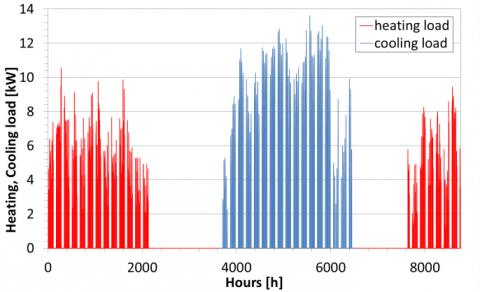Figure 1. Heating and cooling demand on yearly basis

## figure_2.pngFigure 2. Heating and cooling load duration curves

## figure_3.pngFigure 3. Electric load excluding HVAC

The electric load profile, Figure 3, is defined for two type days (weekday, weekend) on the basis of Ref. . The electric annual demand, excluding HVAC requirements, is assumed equal to 52.0 kWh/m2 per year according to an on-site analysis performed on electricity consumption in office buildings .

3. System Lay-Out and Components

To satisfy heating and cooling load of the office a solar electric driven heat pump is here considered as proposed system (PS). The energy conversion system is based on a PV field, an inverter (INV), and a reversible EHP. The PV system covers both electric requirements of the EHP and the end user (lights, PCs, etc.). The system is grid connected and interacts in bidirectional way with external grid. The existing energy conversion system (CS, Conventional System) is based on:

• a natural gas fueled boiler (B) in heating period delivering up to 24.0 kW of thermal power with a thermal efficiency, $\eta_{t h}^{B}$ , of 90.2 %;
• an electric activated chiller (CH), with a cooling power of 13.3 kW and an EER (Energy Efficiency Ratio) equal to 3.0.

In Figure 4 and in Figure 5 heating and cooling operating modes both for proposed and conventional systems are reported.

## figure_4.png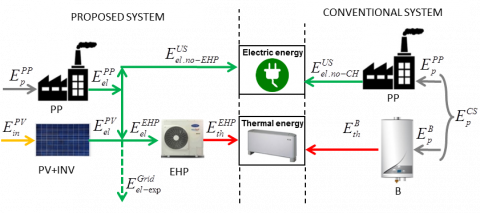Figure 4. Solar PV and CS in heating mode

## figure_5.png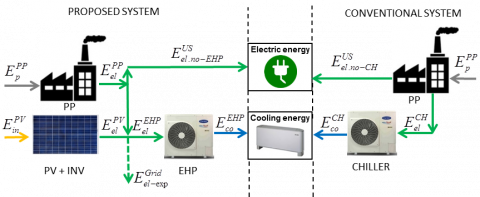Figure 5. Solar PV and CS in cooling mode

Table 2. PV characteristics at STC1

 Max. power [W] 250 Solar panel electric efficiency [%] 15.28 Maximum power voltage [V] 30.38 Maximum power current [A] 8.29 Open circuit voltage, Voc [V] 37.12 Short circuit current, Isc [A] 8.76 Maximum power temperature factor [%/K] -0.42 Temperature coefficient for Voc [%/K] -0.32 Temperature coefficient for Isc [%/K] 0.059 Gross area [m2] 1.64

1 Electrical data evaluated at Standard Test Conditions (STC): air mass 1.5; irradiance equal to 1.0kW/m2; cell temperature equal to 25°C.

In all the considered configurations, the PV panels face south. Furthermore three different peak powers (4500 W, 6000 W, 7500 W) were analyzed. The main characteristic of PV panels are reported in Table 2 .

On Table 3 the inverter characteristics, considered for different peak powers, are shown .

Table 3. Inverter characteristics

 Rated DC input power [W] 5150 6200 7650 Rated AC power [W] 5000 6000 7500 MPPT number [-] 2 2 2 Maximum efficiency [%] 97 97 98 Weighted efficiency (EURO/CEC) [%] 96.4 96.4 97.5 Night tare [W] 0.4 0.4 3

The main data of the reversible air to water heat pump are reported in Table 4 . The EHP provides a nominal heating power of 14.1 kW with a nominal COP (Coefficient of Performance) of 3.19, while rated cooling power achieves 13.3 kW with an EER of 3.32.

Table 4. Air to water heat pump data2

 Heating mode Heating power [kW] 14.1 Electric power input [kW] 4.42 COP [-] 3.19 Cooling mode Cooling power [kW] 13.3 Electric power input [kW] 4.12 EER [-] 3.32

2 Nominal data on the basis of EN 14511:2013 

In cooling period a free cooling system could be activated to reduce the demand. Free cooling air flow rate is 1340 m3/h, and fresh air could be drawn from outside in morning hours (6:30 ÷ 8:00) when external air temperature is below 25 °C.

4. Model Description

TRNSYS is the software considered for the analysis of the system . It is a common used software to perform dynamic simulations of an energy conversion systems used to satisfy energy demand of a building. Each element of the simulated system is modelled through subroutines (so-called “types”) found in the software libraries  . The components can be linked to each other to develop highly structured systems. In the following the models of the main components are briefly analyzed. PV panel are modelled using type 94, , that predicts the current-voltage characteristics of a single module on the basis of a "four-parameter" equivalent circuit built considering manufactures' PV data  . Reversible EHP and CH are modelled on the basis of the performance map of the equipment using type 941 and type 655 , respectively, Natural gas fired boiler is modelled considering constant thermal efficiency by type 6. Finally the building is simulated by type 56 that models the thermal behavior of a building having different thermal zones. The fan coils are simulated as an air to water heat exchanger and are modelled through type 928, in which heating and cooling energy is delivered to an air stream from a source liquid stream .

5. Methodology

In this paragraph a performance analysis of solar system considering the contribution of renewable source on the total space heating and cooling demand is reported. A further study is performed comparing the solar based system (proposed system, PS) with a conventional system (CS) by means of energy, environmental and economic approach.

5.1 Solar system performance analysis

One of the most important parameter to evaluate the performance of the system is the electric efficiency of solar system (SS), $\eta_{e l}^{S S}$  that depends on PV panel efficiency, inverter efficiency and other BOS (Balance Of System) losses (dirt, reflection, cell temperature, wiring, mismatch, etc.) . It reaches on annual basis about 14.6 % for 4500 W and 6000 W while is little higher (14.7 %) for 7500 W. Further interesting parameters, which point out the contribution of renewable source to cover energy demands of the user are heating (SFh), cooling (SFco) and total solar fraction (SFtot), which are respectively defined in Eq. (1), (2) and (3) as :

$S F_{h}=\frac{E_{t h}^{E H P, P V}}{E_{t h}^{U S}}$(1)

$S F_{c o}=\frac{E_{c o}^{E H P, P V}}{E_{c o}^{U S}}$(2)

$S F_{t o t}=\frac{E_{t h}^{E H P, P V}+E_{c O}^{E H P, P V}}{E_{t h}^{U S}+E_{c o}^{U S}}$(3)

where $E_{t h}^{E H P, P V}$  and $E_{c o}^{E H P, P V}$  are, respectively, heating and cooling energy available from EHP evaluated on the basis of electricity contribution due to PV system. If available electricity from PV is used to feed, priority, the EHP. Furthermore $E_{t h}^{U S}$ and $E_{c o}^{U S}$ represent, respectively, space heating and cooling demands.

In Figure 6 and Figure 7 there is the trend of heating and cooling solar fraction varying tilt angle and PV peak power. SFh increases with PV power and tilt angle reducing the contribution needed by electric grid for heating purpose. Its values range between 0.18 and 0.33.

## figure_6.png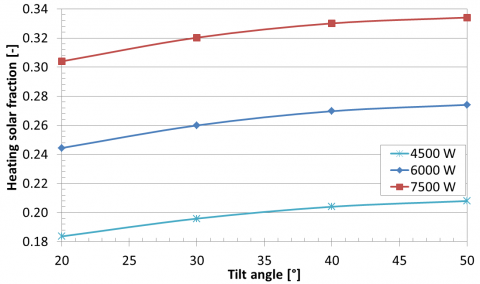Figure 6. Heating solar fraction as a function of PV peak power and tilt angle

Similarly to SFh, SFco increases with PV power while decreases with tilt angle. This parameter shows higher values than SFh due to increased availability of solar radiation during summer period. Its value achieves 0.84 for 7500 W and 20°.

## figure_7.pngFigure 7. Cooling solar fraction as a function of PV peak power and tilt angle

Finally SFtot, as expected, increases with PV power while shows a maximum for about 29°. For this angle SFtot ranges between 0.40 for 4500 W and 0.61 for 7500 W, meaning for the last case, that 61 % of space heating and cooling demand is satisfied by renewable energy.

## figure_8.png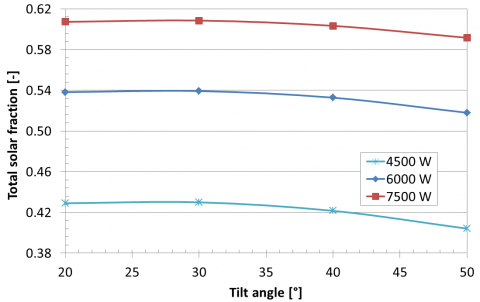Figure 8. Total solar fraction depending on PV peak power and tilt angle

5.2 Energy, environmental and economic analysis

The performance of the solar based system used to meet electric, heating and cooling demand are compared with those of a reference system based on an existing natural gas-fired boiler and electric chiller, excluding in this case free cooling option.

5.2.1 Energy analysis

The performance of the analyzed systems have been estimated by means of Fuel Energy Saving Ratio (FESR), comparing the primary energy consumption due to fossil fuel of proposed ( $E_{p}^{P S}$ ) and conventional ( $E_{p}^{C S}$ ) systems, as shown in Figure 4 and in Figure 5. Electric reference efficiency ( $\eta_{e l}^{P P}$ ) is considered equal to 41.3 %, which is evaluated on the basis of average Italian thermo-electric power plant electric efficiency that includes transmission and distribution grid losses . FESR could be defined as:

$F E S R=\frac{E_{p}^{C S}-E_{p}^{P S}}{E_{p}^{C S}}$(4)

where, with i = 1 (heating), 2 (cooling), 3 (intermediate):

$E_{p}^{C S}=E_{p}^{P P}+E_{p}^{B}=\sum_{i=1}^{3}\left(\frac{E_{e l, n o-C H}^{U S}}{\eta_{e l}^{P P}}\right)_{i}+\frac{E_{e l}^{C H}}{\eta_{e l}^{P P}}+\frac{E_{t h}^{B}}{\eta_{t h}^{B}}$(5)

$E_{p}^{P S}=E_{p}^{P P}-E_{p}^{G r i d}=\sum_{i=1}^{3}\left(\frac{E_{e l}^{P P}-E_{e l-e x p}^{G r i d}}{\eta_{e l}^{P P}}\right)_{i}$(6)

In particular, as stated in Eq. (5), primary energy of CS depends on electric energy required for cooling equipment, $E_{e l}^{C H}$ , non HVAC demand (lighting, appliances, etc.), $E_{e l, n o-C H}^{U S}$ , and thermal energy satisfied by natural gas boiler, $E_{t h}^{B}$  An equivalent equation could be introduced for PS, even if it depends on the electric energy drawn from the grid ( $E_{e l}^{P P}$ ) and on the electricity exported to the grid ( $E_{e l-e x p}^{G r i d}$ ), that is considered as a credit for primary energy evaluation. In Figure 9 FESR as a function of PV peak power and tilt angle is shown. FESR has an increasing trend with the peak power thanks to the greater availability of renewable electric energy. The FESR is always greater than 48 % and its maximum (81.2 %) is achieved for a tilt angle of 31° and 7500 W.

## figure_9.png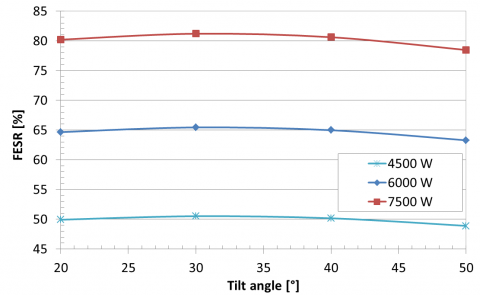Figure 9. FESR as a function of PV peak power and tilt angle

One of the main issues related to PV plants is the exported electricity that could lead to problems on electric grid. In this way it could be important to evaluate, on the basis of electric energy required by end user the best configuration characterized by the lowest percentage of electricity exported. Total electric energy, including also EHP, required by end user for PS is 13.7 MWh. Considering the best configuration of each peak power, characterized by a tilt angle of 31°, electric energy available from PV is partly used by the end user and partly exported to the grid, Figure 10. Two indexes could be introduced to highlight the self-consumption of PV electricity, Table 5:

• RPVtoUS/US-tot: ratio between electric energy supplied by PV to end user and the total one that it requires;
• RPVtoUS/PV-tot: ratio between electricity delivered by PV to end user and total one available from PV.

On Table 5 total solar radiation that reaches the PV panels and also electricity production are reported. Increasing the size of PV system renewable electricity covering end user demand increases achieving 48.6 % for 7500 W. Considering the fraction of PV electricity self-consumed, with respect to global production, the trend is different leading to an increased export of energy to the grid with PV size.

## figure_10.png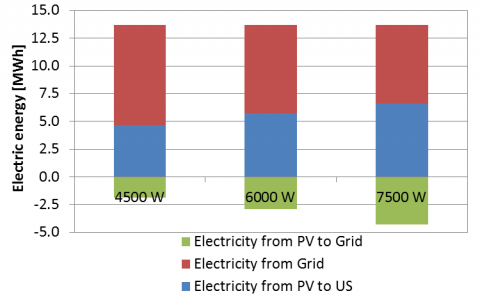Figure10. Electric energy distribution as a function of PV peak power for 31°

Table 5. Ratio of electricity from PV

 PV power [W] 4500 6000 7500 Total solar radiation [MWh] 44.7 59.7 74.6 Electricity from PV [MWh] 6.51 8.69 10.94 RPVtoUS/US-tot [%] 33.8 42 48.6 RPVtoUS/PV-tot [%] 71.1 66.3 60.7
In order to reduce exported electricity an electric battery could be considered. A further analysis could be performed considering the linear decreasing performance per year of PV system leading to an electric energy reduction availability, at 25th year, of 17.4 % . In Figure 11, for a tilt angle of 31°, FESR as a function of PV peak power and operating year is shown. In the best case, characterized by 7500 W, FESR shows a reduction to 68.1 %.

## figure_11.png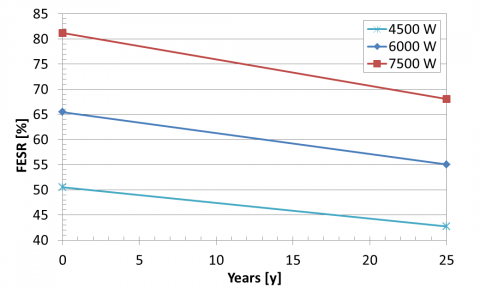Figure 11. FESR as a function of PV peak power and operating year for 31°

5.2.2 Environmental analysis

The environmental performance is based on a simplified approach by means of equivalent carbon dioxide emissions (CO2). The analysis is performed comparing the avoided CO2 emissions of the proposed system ( $C O_{2}^{P S}$ ) with the reference one ( $\mathrm{CO}_{2}^{\mathrm{CS}}$ ). The CO2 emissions for each system are evaluated introducing CO2 emission factors: natural gas factor, β, is equal to 0.205 kg of CO2 for each kWh of primary energy related to the input fuel, while the electricity factor, α, is 0.563 kg for each kWh of electric energy drawn from the grid considering the average Italian emissions of the thermo-electric plants mix that includes grid losses . Similarly to FESR, ∆CO2 is defined as:

$\Delta \mathrm{CO}_{2}=\frac{\mathrm{CO}_{2}^{C S}-\mathrm{CO}_{2}^{P S}}{\mathrm{CO}_{2}^{C S}}$(7)

where, with i = 1 (heating), 2 (cooling), 3 (intermediate):

$C O_{2}^{C S}=C O_{2}^{P P}+C O_{2}^{B}=\alpha \cdot \sum_{i=1}^{3}\left(E_{e l, n o-C H}^{U S}\right)_{i}+\alpha \cdot E_{e l}^{C H}++\beta \cdot E_{p}^{B}$(8)

$C O_{2}^{P S}=C O_{2}^{P P}-C O_{2}^{G r i d}=\alpha \cdot \sum_{i=1}^{3}\left(E_{e l}^{P P}-E_{e l-e x p}^{G r i d}\right)_{i}$(9)

CO2 emission related to electricity exported to the grid is considered as a credit for $C O_{2}^{P S}$  evaluation. ∆CO2 has a trend that is similar to FESR achieving a maximum for an angle of 31° for each peak power, Table 6.

Table 6. ΔCO2 as a function of PV power, tilt angle 31°

 PV power [W] 4500 6000 7500 ΔCO2 [%] 49.7 64.9 80.9

5.2.3 Economic analysis

The economic analysis was carried out starting by operating and investment costs of proposed and conventional systems. In particular a unit natural gas price, cu,NG, equal to 0.90 €/Nm3, a unit electricity price for purchased electricity, cu,el, of 0.20 €/kWh both for PS and CS is considered. A feed-in tariff, cu,el-exp, equal to 0.11 €/kWh for sold electricity and an annual ordinary maintenance cost of 18.5 € per kW of peak PV power are here considered . A specific investment cost decreasing with plant size in the range 2224 €/kW (4500 W) to 2179 €/kW (7500 W), that includes PV plant components (panels, inverter, cables, etc.), flat roof PV frame, transportation, installation, design and 10 % in terms of VAT, is here considered. Finally an investment cost for EHP, ICEHP, of 6217 € is introduced. Economic analysis of a solar heat pump system, could be based using different methodologies, such as net present value, annualized life cycle cost, internal rate of return, etc.. In this study a simplified approach using the simple payback period, SPB, index is considered. This index estimates the payback period of an investment and is defined as:

$S P B=\frac{I C}{\sum_{j=1}^{N} F_{j}}$(10)

$I C=I C^{S S}+I C^{E H P}$(11)

$F_{j}=\Delta O C_{j}=O C_{j}^{C S}-O C_{j}^{P S}$(12)

$O C_{j}^{C S}=V O_{N G} \cdot c_{u, N G}+\left(E_{e l}^{C H}+E_{e l, n o-C H}^{U S}\right) \cdot c_{u, e l}$(13)

$O C_{j}^{P S}=E_{e l}^{P P} \cdot c_{u, e l}+O C_{m a n}^{P V}-E_{e l-e x p}^{G r i d} \cdot c_{u, e l-e x p}$(14)

considering that:

• IC is the investment cost of the PS (PV plant, EHP);
• ICSS is the total investment cost of PV plant;
• Fj represents the cash flow for the generic year j;
• $O C_{j}^{P S}$  and $O C_{j}^{c s}$ are the operating costs of the proposed and conventional systems;
• ΔOCj is the difference between the operating costs of the proposed and conventional systems;
• $O C_{\operatorname{man}}^{P V}$  is the maintenance cost for PV system;
• VONG is the volume of fuel (natural gas) used to operate the boiler estimating a lower heating value of 9.52 kWh/Nm3.

Similarly to energy and environmental analysis, operating cost savings, ΔOC, due to introduction of PS increases with PV peak power reaching the maximum for a tilt angle of 31° for each configuration, Figure 12.

Operating costs related to electricity, space heating and cooling for CS are about 2965 € (2525 € for electricity and 440 € for natural gas). The evaluation of SPB could be based on two different scenarios, Table 7:

• grid parity: characterized by an IC without any economic support;
• support action: Italian government gives incentives covering 50 % of IC both for PV and EHP .

## figure_12.png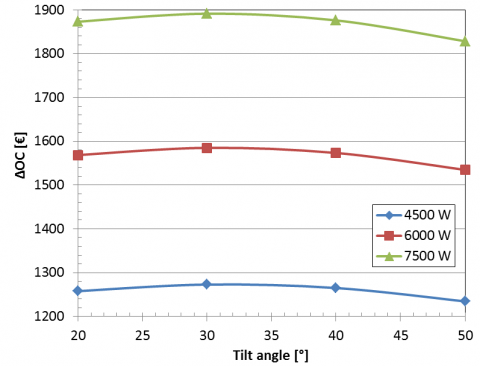Figure 12. Annual operating costs difference as a function of PV power and tilt angle

Table 7. SPB without and with economic support

 PV power [W] SPB in grid parity [y] SPB with 50% support [y] 4500 12.8 6.4 6000 12.4 6.2 7500 12.0 6.0

In presence of economic support action the economic analysis leads to interesting results with SPB lower than 7 years for each configuration. In Figure 13 the SPB varying electricity and natural gas unit prices is shown, considering for the configuration characterized by the lowest exported electricity (4500 W, 31° tilt angle), the presence of support action (50 % of IC), and a fixed unitary feed-in tariff equal to 0.11 €/kWh for exported electricity. SPB shows a decrease with the cost of electricity and natural gas. For high electricity and gas costs this index may decrease to values close to 5 years.

## figure_13.png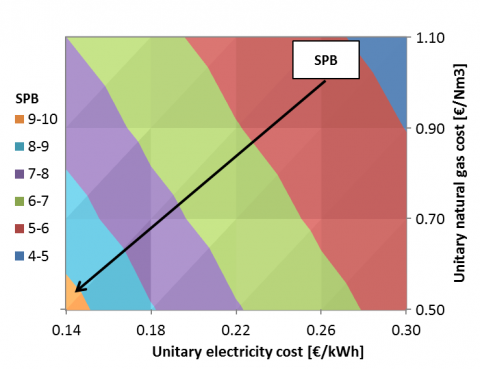Figure 13. SPB as a function of unitary price of electricity and natural gas

6. Conclusions

A solar electric driven heat pump, based on an EHP interacting with PV field, to meet electric, thermal and cooling demand of an office building located in Naples is here considered. Dynamic simulations were performed to assess the energy, economic and environmental performance of the proposed system varying some parameters (PV peak power, tilt angle, etc.). Yearly PV plant efficiency, including inverter and PV efficiency and also other BOS losses, is in the range between 14.6 % (4500 W, 6000 W) and 14.7 % (7500 W). Total solar fraction reaches 0.43 for 4500 W and 0.61 for 7500 W for a tilt angle of 29°. Similar results were obtained for the energy and environmental impact analysis. FESR and ∆CO2 are always higher than 48 % with a maximum of about 81 % for 7500 W and a tilt angle of 31°. In this last configuration, even if FESR shows a reduction depending on operating year, it remains higher than 68.1 % after 25 years. Increasing the size of PV system, renewable electricity covering total demand achieves 48.6 %, while the fraction exported increases up to 39.3 % for 7500 W. To decrease electricity exported an electric battery could be considered to reduce the interaction with electric grid. The economic analysis reveals a decrease of operating costs, even if investment costs, due to PV field and EHP, highlight the need of government incentives that could lead to interesting findings for such technology. A SPB lower than 7 years for each configuration considered could be achieved. The result could be improved in case of increase of electricity and natural gas prices.

Nomenclature
 AC Alternate Current B Boiler BOS Balance Of System cu Specific electricity cost/reward and natural gas cost, €/kWh, €/Nm3 CH Chiller CO2 Equivalent dioxide carbon emission, kg CO2/y COP Coefficient Of Performance, - CS Conventional System DC Direct Current E Energy, kWh/y EER Energy Efficiency Ratio, - EHP Electric Heat Pump F Cash flow, €/y GHP Gas Heat Pump GSHP Ground Source Heat Pump HVAC Heating and Ventilation Air Conditioning I Current, A IC Investment Cost, € INV Inverter MPPT Maximum Power Point Tracker OC Operating Cost, €/y PP Power Plant PS Proposed System PV Photovoltaic SF Solar Fraction, - SHC Solar Heating and Cooling SPB Simple Pay Back SS Solar System STC Standard Test Conditions US End User V Voltage, V VAT Value-Added Tax VO Volume, Nm3 Greek symbols α Emission factor for electricity, kg CO2/kWhel β Emission factor for natural gas, kg CO2/kWhEp η Efficiency, -, % Δ Difference Subscripts co cooling el electric el,no-CH electric excluding chiller el,no-EHP electric excluding EHP el-exp electric exported h heating man maintenance NG Natural Gas p primary th thermal tot total
References

 European Union. (2015). EU energy in figures. Statistical pocketbook 2015. Publications office of the European Union. Luxembourg. [Online]. Available: ec.europa.eu/energy/sites/ener/files/documents/PocketBook_ENERGY_2015 PDF final.pdf

 European Union. (2013). EU energy in figures. Statistical pocketbook 2013. Publications office of the European Union. Luxembourg. [Online]. Available: ec.europa.eu/energy/sites/ener/files/documents/2013_pocketbook.pdf

 X. Wang and M. Dennis, “Influencing factors on the energy saving performance of battery storage and phase change cold storage in a PV cooling system,” Energy Build, vol. 107, pp. 84-92, 2015. DOI: 10.1016/j.enbuild.2015.08.008.

 L. Ni, D. Qv, Y. Yao, F. Niu and W. Hu, “An experimental study on performance enhancement of a PCM based solar-assisted air source heat pump system under cooling modes,” Appl Therm Eng, vol. 100, pp. 434-452, 2016. DOI: 10.1016/j.applthermaleng.2016.02.001.

 F. Khalid, I. Dincer and M. A. Rosen, “Techno-economic assessment of a renewable energy based integrated multigeneration system for green buildings,” Appl Therm Eng, vol. 99, pp. 1286-1294, 2016. DOI: 10.1016/j.applthermaleng.2016.01.055.

 F. Calise, M. Dentice d'Accadia and A. Piacentino, “A novel solar trigeneration system integrating PVT (photovoltaic/thermal collectors) and SW (seawater) desalination: Dynamic simulation and economic assessment,” Energy, vol. 67, pp. 129-148, 2014. DOI: 10.1016/j.energy.2013.12.060.

 I. Sarbu and Sebarchievici C., “Review of solar refrigeration and cooling systems,” Energy Build, vol. 67, pp. 286-297, 2013. DOI: 10.1016/j.enbuild.2013.08.022.

 T. X. Li, R. Z. Wang and H. Li, “Progress in the development of solid-gas sorption refrigeration thermodynamic cycle driven by low-grade thermal energy,” Prog Energ Combust, vol. 40, pp. 1-58, 2014. DOI: 10.1016/j.pecs.2013.09.002.

 R. Sekret and M. Turski, “Research on an adsorption cooling system supplied by solar energy,” Energy Build, vol. 51, pp. 15-20, 2012. DOI: 10.1016/j.enbuild.2012.04.008.

 B. Tashtoush, A. Alshare and S. Al-Rifai, “Hourly dynamic simulation of solar ejector cooling system using TRNSYS for Jordanian climate,” Energ convers manage, vol. 100, pp. 288-299, 2015. DOI: 10.1016/j.enconman.2015.05.010.

 G. Angrisani, C. Roselli, M. Sasso and F. Tariello, “Assessment of energy, environmental and economic performance of a solar desiccant cooling system with different collector types,” Energies, vol. 7, pp. 6741-6764, 2014. DOI: 10.3390/en7106741.

 C. A. Balaras, G. Grossman, H. M. Henning, C. A. Infante Ferreira, E. Podesser, L. Wang and E. Wiemken, “Solar air conditioning in Europe-an overview,” Renew Sust Energ Rev, vol. 11, pp. 299-314, 2007. DOI: 10.1016/j.rser.2005.02.003.

 A. de Geus, H. de Beijer and L. Krosse, “The SolabCool®, cooling of dwellings and small offices by using waste or solar heat,” Energy Procedia, vol. 70, pp. 23-31, 2015. DOI: 10.1016/j.egypro.2015.02.093.

 M. Izquierdo, P. de Agustín and E. Martín, “A micro photovoltaic-heat pump system for house heating by radiant floor: some experimental results,” Energy Procedia, vol. 48, pp. 865-875, 2014. DOI: 10.1016/j.egypro.2014.02.100.

 N. Hartmann, C. Glueck and F. P. Schmidt, “Solar cooling for small office buildings: Comparison of solar thermal and photovoltaic options for two different European climates,” Renew Energ, vol. 36, pp. 1329-1338, 2011. DOI: 10.1016/j.renene.2010.11.006.

 A. Franco and F. Fantozzi, “Experimental analysis of a self-consumption strategy for residential building: The integration of PV system and geothermal heat pump,” Renew Energ, vol. 86, pp. 1075-1085, 2016. DOI: 10.1016/j.renene.2015.09.030.

 F. Reda, N. Arcuri, P. Loiacono and D. Mazzeo, “Energy assessment of solar technologies coupled with a ground source heat pump system for residential energy supply in Southern European climates,” Energy, vol. 91, pp. 294-305, 2015. DOI: 10.1016/j.energy.2015.08.040.

 Ergonomics of the thermal environment - Analytical determination and interpretation of thermal comfort using calculation of the PMV and PPD indices and local thermal comfort criteria, ISO 7730:2005, 2005.

 2011 ASHRAE Handbook-Fundamentals, ASHRAE Inc., USA, 2011.

 Italian Legislative Decree n. 59 of April 2, 2009.

 P. Caputo, G. Costa, V. Canotto, L. Pistocchini and A. Roscetti. (2011, July). Platform behavior in relation to the proper combination of demand curves and generation and control systems. ENEA, Italy. [Online]. Available:www.enea.it/it/Ricerca_sviluppo/documenti/ricerca-di-sistema-elettrico/efficienza-energetica-servizi/rds-55.pdf

 E. Gruber, S. Plesser, R. Dusèe, I. Sofronis, P. Lima, Rivière and A. Rialhe. (2008, August). EL-TERTIARY Monitoring Electricity Consumption in the Tertiary Sector, Intelligent Energy Europe. [Online]. Available: http://ec.europa.eu/energy/intelligent/projects/en/printpdf/projects/el-tertiary

 Conergy, www.conergy.com, datasheet PowerPlus 250P.

 ABB, new.abb.com/power-converters-inverters/it/solare, datasheet Solar inverter.

 Aermec, www.aermec.com, datasheet ANL 050 H.

 Parts 1 – 4 Air conditioners, liquid chilling packages and heat pumps with electrically driven compressors for space heating and cooling , EN 14511:2013, 2013.

 H.-M. Henning and J. Döll, “Solar systems for heating and cooling of buildings,” Energy Procedia, vol. 30, pp. 633-653, 2012. DOI: 10.1016/j.egypro.2012.11.073.

 TRNSYS 17, a TRaNsient SYstem Simulation program, Solar Energy Laboratory, University of Wisconsin-Madison, 2010.

 T.E.S.S. Component Libraries v.17.01 for TRNSYS v17.0 and the TRNSYS Simulation Studio, Parameter/Input/Output Reference Manual, Thermal Energy System Specialists, LLC, 2004.

 D. Mazzeo, N. Matera, P. Bevilacqua and N. Arcuri, “Energy and economic analysis of solar photovoltaic plants located at the University of Calabria”, International Journal of Heat and Technology, vol. 33, pp. 41-50, 2015. DOI: 10.18280/ijht.330406.

 T. U. Townsend, “A Method for Estimating the long-term performance of direct-coupled photovoltaic systems,” M.S. Thesis, Solar Energy Laboratory, University of Wisconsin, Madison, 1989.

 J. A. Duffie and A. B. William. “Solar engineering of thermal processes,” John Wiley & Sons, Inc., New York, USA, 1991.

 E. Entchev, P. Tzscheutschler, K. Darkovic, M. Sasso, A. Hawkes, A. Akisawa and C. Roselli. (2014, October). Integration of Microgeneration and Related Technologies in Building, Final Report of Annex 54. International Energy Agency, [Online]. Available: www.iea-ebc.org/fileadmin/user_upload/docs/Annex/EBC_Annex_54_Micro-Generation_Integration_Final_Report.pdf

 Sabiana, www.sabiana.it, datasheet CRC 43.

 A. Malara, C. Marino, A. Nucara, M. Pietrafesa, F. Scopelliti and G. Streva, “Energetic and economic analysis of shading effects on PV panels energy production,” International Journal of Heat and Technology, vol. 34, pp. 465-472, 2016. DOI: 10.18280/ijht.340316.

 TERNA. (2012). Statistics and forecasts, Statistical data, Production. [Online]. Available: download.terna.it/terna/0000/0525/58.pdf

 ISPRA, CO2 emission factors in electricity sector and analysis of emissions decomposition, [Online]. Available:www.isprambiente.gov.it/files/pubblicazioni/rapporti/rapporto_172_2012.pdf

 National Renewable Energy Laboratory, NREL, Department Of Energy, USA, Distributed Generation Renewable Energy Estimate of Costs, 2016, www.nrel.gov/analysis/tech_lcoe_re_cost_est.html

 Italian Legislative Decree n. 208 of December 28, 2015.